# 1.本周学习总结（0-5分）## 1.1 查找的性能指标

• ASL成功：找到某一记录合集中任一记录平均所要的关键字比较的次数。
• ASL不成功：在某记录合集中找不到某一记录平均所要的关键字比较次数。
例：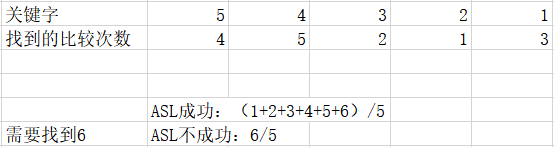• 比较次数：找到或者找不到某一记录的次数
• 移动次数：例如哈希表和哈希链有冲突时需要移位找到空的位置所需要的次数
• 时间复杂度：顺序查找：时间复杂度O(N)，二分查找：时间复杂度O(logN)

## 1.2 静态查找

• 顺序查找
顺序查找：从表的一端开始，顺序扫描线性表，将扫描到的关键字与给定的K值相比较。当前扫描到的关键字与k相等，则查找成功，如果扫描结束后仍未找到关键字，等于K的记录则查找失败。
ASL成功=(n+1)/2
ASL不成功=n
平均时间复杂度:O(n)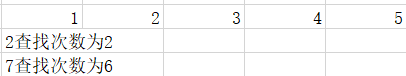• 二分查找
二分查找要求线性表示有序表，以折半的方式进行查找
二分查找，时间复杂度O(logN)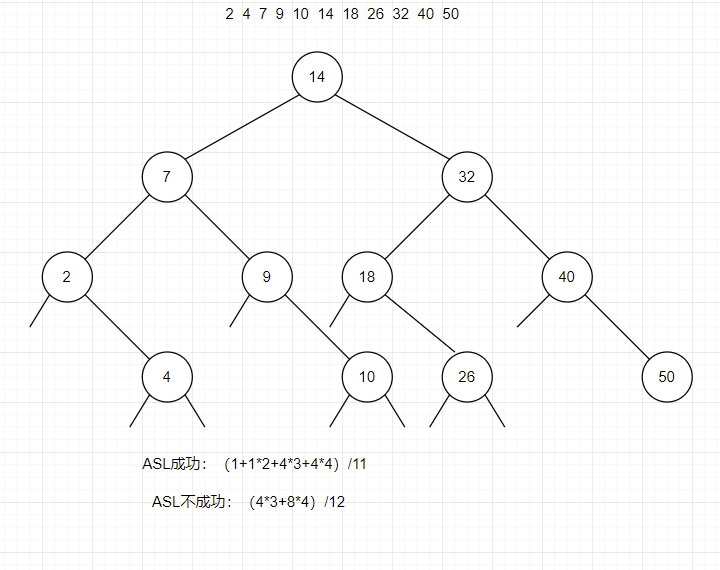ASL成功=（对应层数 * 对应层的结点个数，进行累加）/总的结点个数
ASL不成功=（对应层数 * 对应层的空结点个数，进行累加）/总的空结点个数

## 1.3 二叉搜索树

### 1.3.1 如何构建二叉搜索树（操作）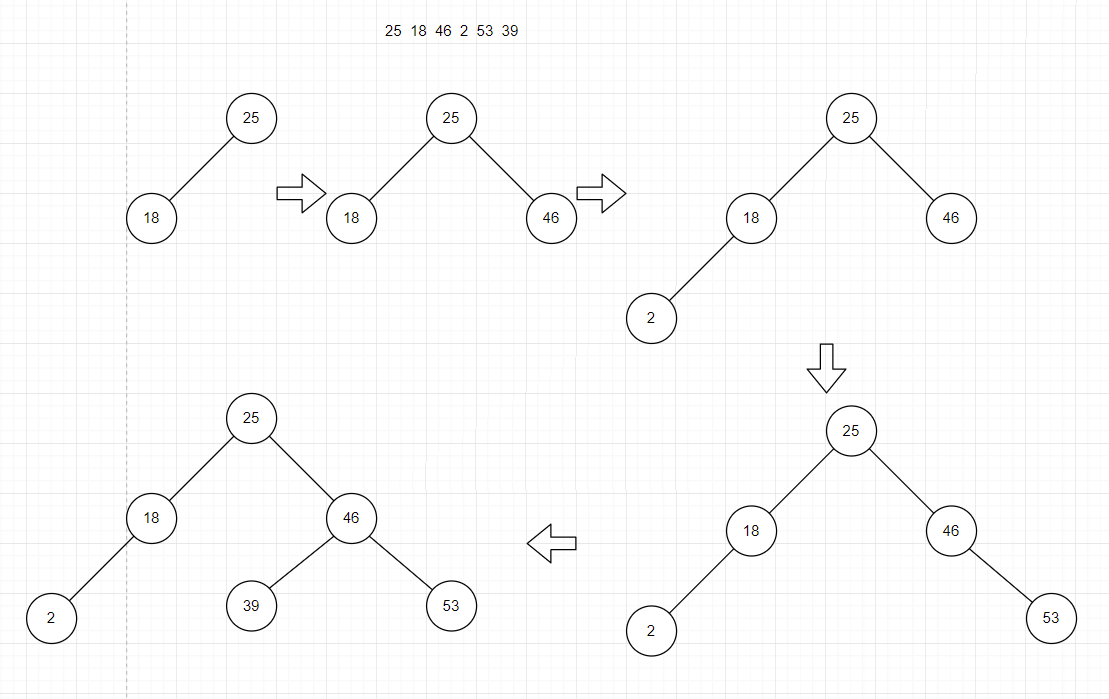ASL成功=（1+2 * 2 + 3 * 3）/6
ASL不成功=(1 * 2 + 6 * 3)/7
ASL成功=（对应层数 * 对应层的结点个数，进行累加）/总的结点个数
ASL不成功=（对应层数 * 对应层的空结点个数，进行累加）/总的空结点个数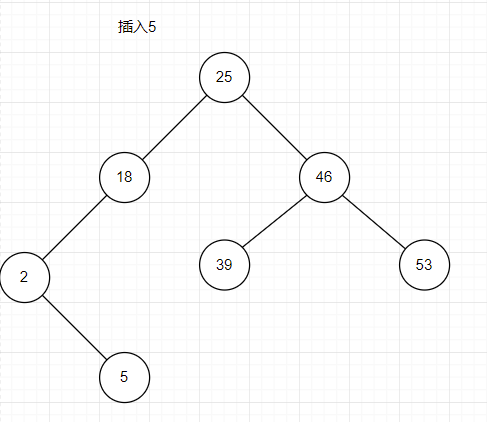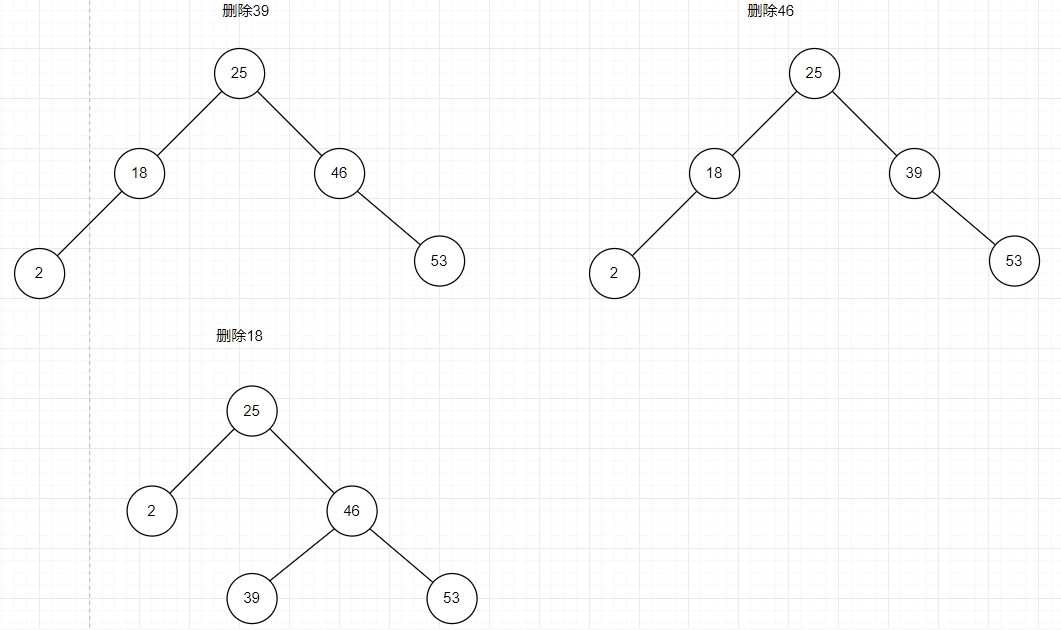### 1.3.2 如何构建二叉搜索树（代码）

• 构建及构建：
void Insert(BinTree &T, int a)
{
if (T == NULL)//该结点为空则新建
{
T = new TNode;
T->data = a;
T->left = NULL;
T->right = NULL;
}
else
{
if (a > T->data)//进入右递归
{
return Insert(T->right, a);
}
else if (a < T->data)//进入左递归
{
return Insert(T->left, a);
}
}
}


BinTree Delete( BinTree BST, ElementType X )
{
BinTree p;
if (!BST )//树为空
{
return BST;
}

if ( BST->Data > X)//进入左递归查找
{
BST->Left = Delete( BST->Left, X);
}
else if ( BST->Data < X)//进入右递归查找
{
BST->Right = Delete( BST->Right, X);
}
else
{
if ( BST->Right && BST->Left )
{
p = FindMax( BST->Left );//找左子树的最大结点
BST->Data = p->Data;//替代
BST->Left = Delete( BST->Left, BST->Data );//删除左子树的最大结点
}
else
{
p = BST;
if ( !BST->Left )//左子树为空
{
BST = BST->Right;
}
else if ( !BST->Right)//右子树为空
{
BST = BST->Left;
}
free(p);
}
}
return BST;
}


## 1.4 AVL树

AVL树可适当减少树的高度，减少查找次数，优化其结构，减少时间复杂度，提高运行效率。

• LL型调整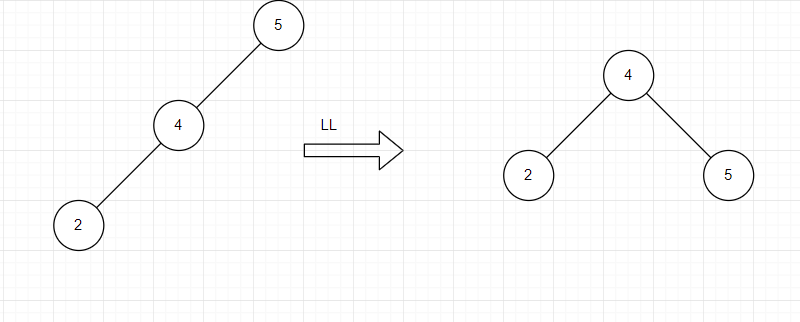• RR型调整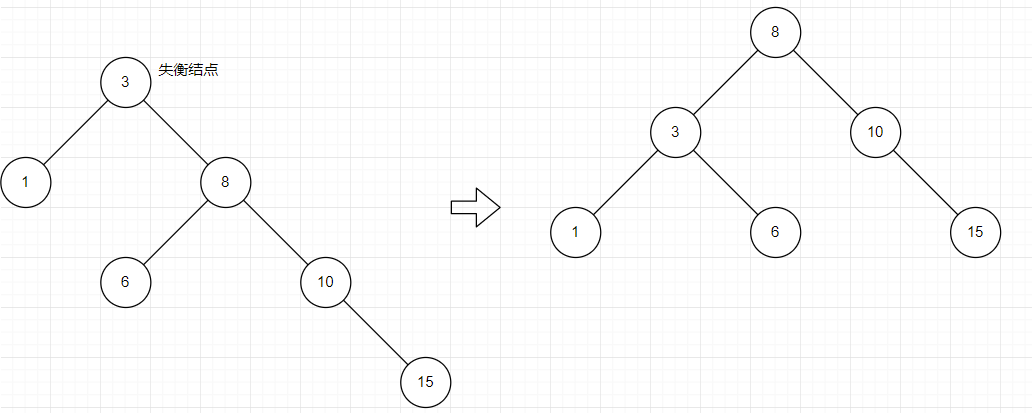• LR型调整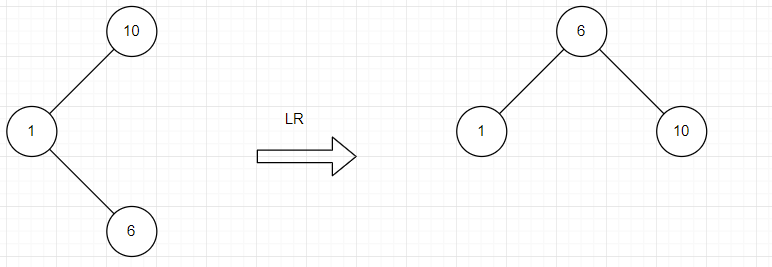• RL型调整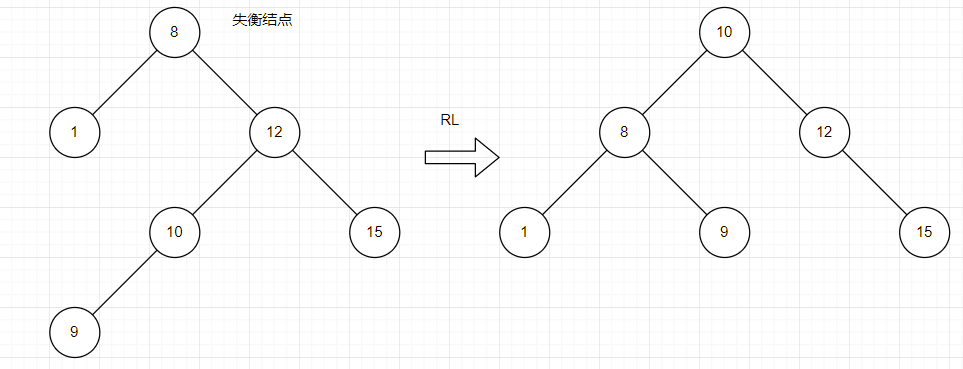AVL树结点个数最少满足的条件：O(1)=1,O(2)=2,O(H)=O(H-1)+O(H-2)+1
n个结点的AVL树满足:h=log2(N(h)+1)

#### map用法

#include <map>  //STL头文件没有扩展名.h


方法一insert插入

users.insert(pair<long long, string>(num, code));
users.insert(pair<long long, string>(10, 5))

user=code;
user='5'


map<long long, string>users;
map<long long, string>::iterator iter;
iter = users.find(num);
if (iter == users.end())//到达结尾没找到
{
证明没找到;
}


clear(）        删除所有元素
empty()         如果map为空则返回true
erase()         删除一个元素
find()          查找一个元素
insert()        插入元素
size()          返回map中元素的个数


## 1.5 B-树和B+树

• B-树
AVL由于一个结点只能存放一个关键字，若数据量太多会导致树的高度过高，B树的一个结点则可存放多个关键字，降低树的高度，减少查找时间。
一个m阶的B-树每个结点最多m个孩子结点，最多m-1个关键字。除了根节点外，其他结点至少m/2个孩子结点，至少m/2-1个关键字。根节点至少2个孩子。
B-树的查找是通过定义结点的孩子指针个数，通过比较该节点中的关键字，找到对应的孩子指针所指的元素，继续进行比较。

B-树的插入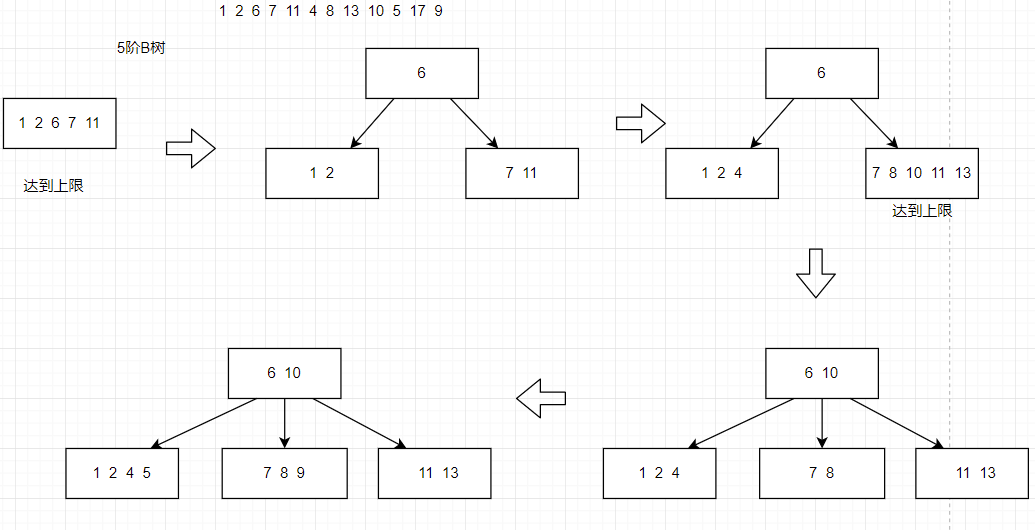B-树的删除• B+树
与B-树的区别，若其结点有n棵子树则有n个关键字，所有的叶子结点中包含了全部关键字的信息，以及指向含这些关键字记录的指针，叶子结点本身依关键字的大小自小而则顶序链接。
可解决索引块系统一类的功能，例如文件查找索引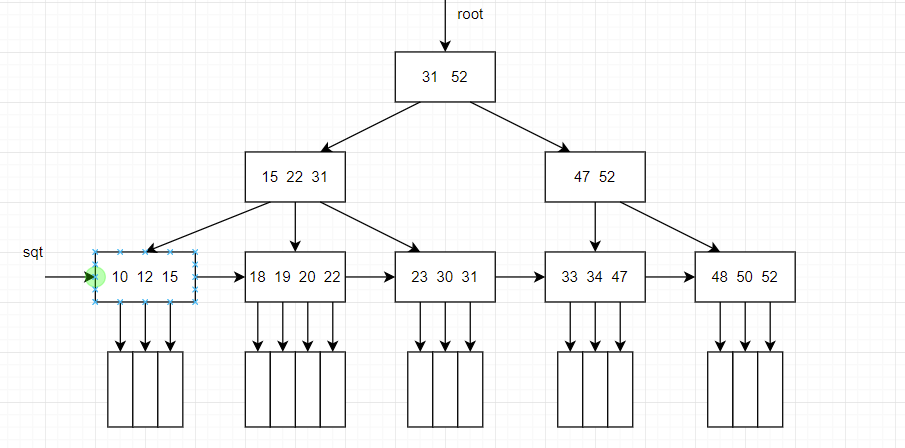## 1.6 散列查找

• 哈希表
利用除留余数法确定对应的下标，当该位置已有元素时，自动往后一位移动，直至遇到空位，探测次数累次增加。
例题：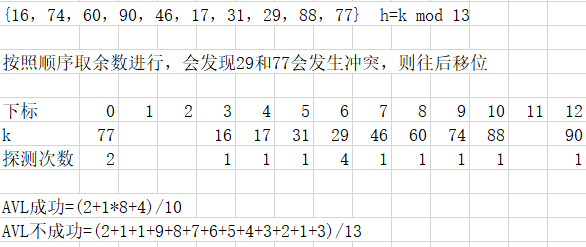AVL成功=总的探测次数和/有效元素个数
AVL不成功=（从0开始到第p个单元格的不成功探测次数和，例如p为7，但建了大于7的单元格，只要计算0到6的单元格不成功探测次数即可）/p

• 哈希链
同样除留余数法确定对应的下标，但由于是建了链，当对应下标的链有元素时，采用头插法插入其中。
例题：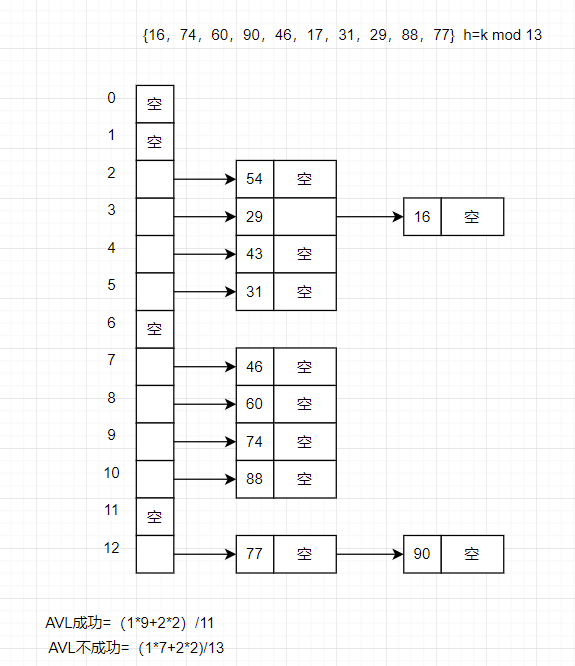AVL成功=（按层来看，一列元素为一层，向外扩张。层数 * 对应层数的元素个数总和）/总元素个数
AVL不成功=元素总个数/p

# 2.PTA题目介绍（0--5分）

## 2.1 是否完全二叉搜索树（2分）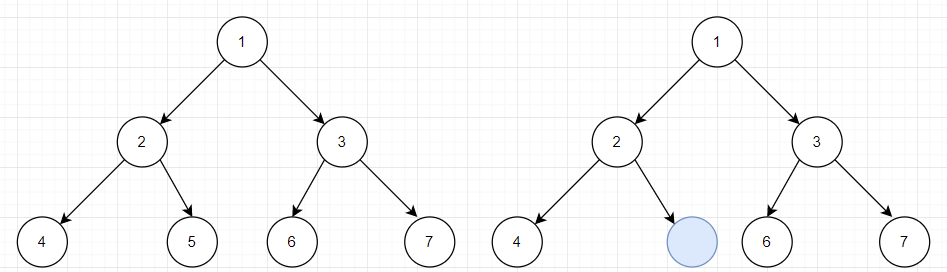### 2.1.1 伪代码

/*建立平衡二叉树*/
void Insert(BinTree &T, int a)
{
if (结点为空)
{
新建结并且初始化;
}
else
{
if (a < T值)进入右循环;
if (a > T值)进入做循环;
}
}
/*层次遍历*/
int falg = 0;
q.push(T);
while (!q.empty())
{
if (遇到空结点)
{
flag = 1;
}
else
{
if (flag = 0)进行计数;
左孩子入队;
右孩子入队;
}
}


### 2.1.2 提交列表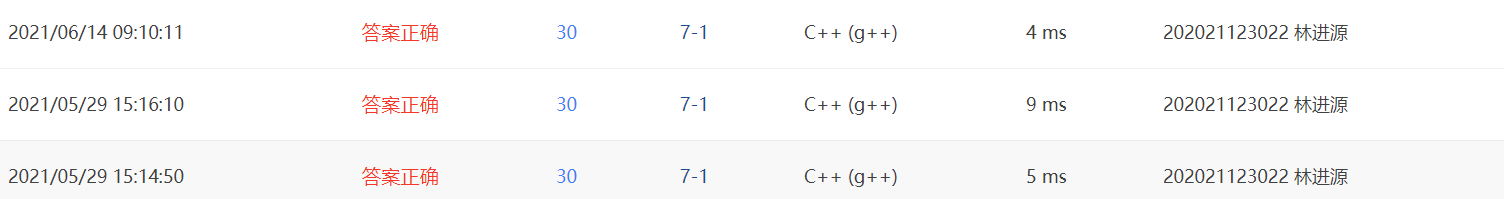## 2.2 航空公司VIP客户查询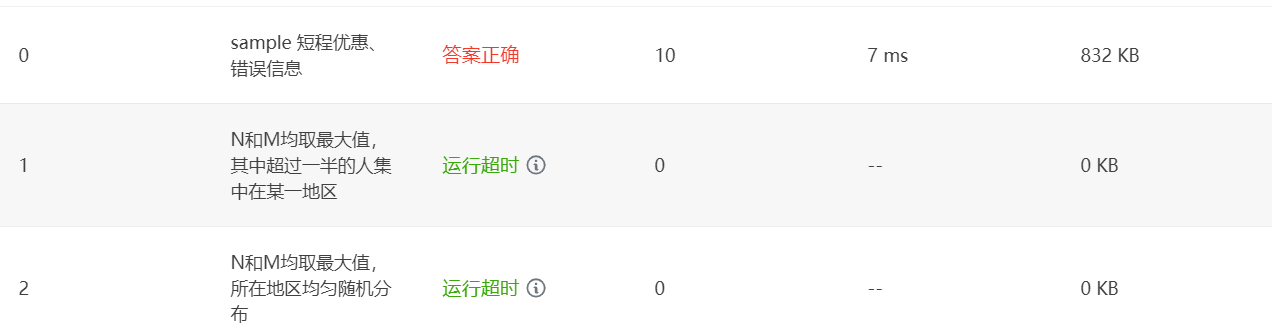### 2.2.1 伪代码

/*结构体*/
typedef struct node
{
char id;
int distance;
struct Tnode *next;
}HashTnode,*HashList;

/*取余求地址*/
for (int i = 12; i <= 16; i++)
{
换成数据;
}
/*查找用户是否已存在*/
while (遍历哈希链)
{
比较用户名;
}
/*存放数据*/
if (用户存在)
{
比较路程;
存放数据;
}
else
{
新建结点;
比较路程存放数据;
}


### 2.2.2 提交列表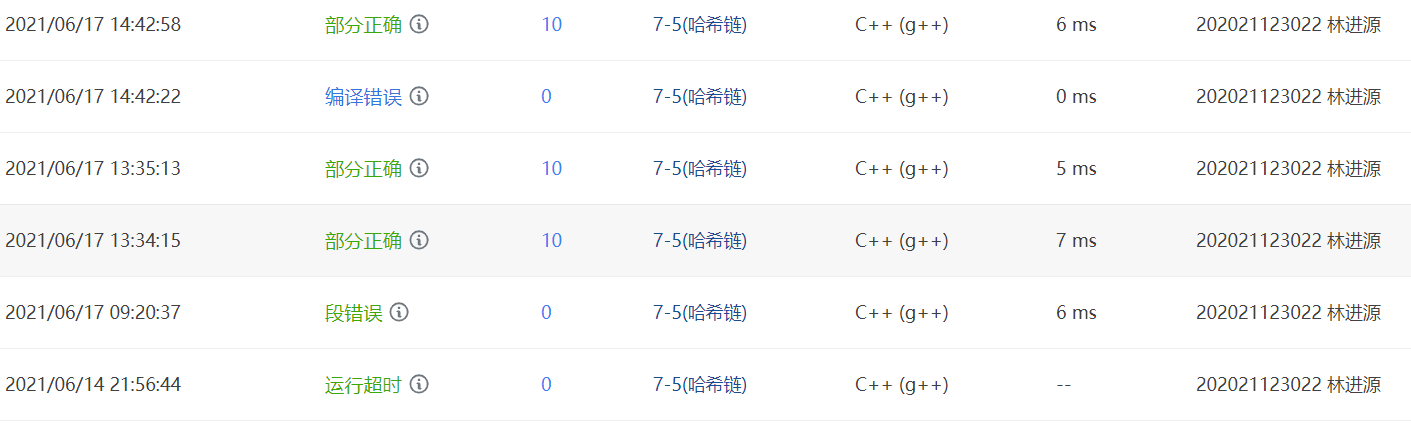## 2.3基于词频的文件相似度

### 2.3.1 伪代码

map<string, bool>m;//如果第i个文件存在单词word，则m[i][word] = true;
static int same;//定义二维数组存放是否有相似
static int num;

for (到达文件个数)
{
while (直到遇到'#')
{
全部字母变为大写;
if (字母个数小于10)
逐个放入数组;
if (字母总个数大于3)
则map[i][word] = true;
}
for (it = m[i].begin(); it != m[i].end(); it++)
{
for (j = 0; j <= i; j++)
{
if (m[j][it->first] == true)//i中的词组在j中出现
same[i][j] = same[j][i] += 1;
}
}
记录第i个文件的词组总数放入num[i]中;
}



### 2.3.2提交列表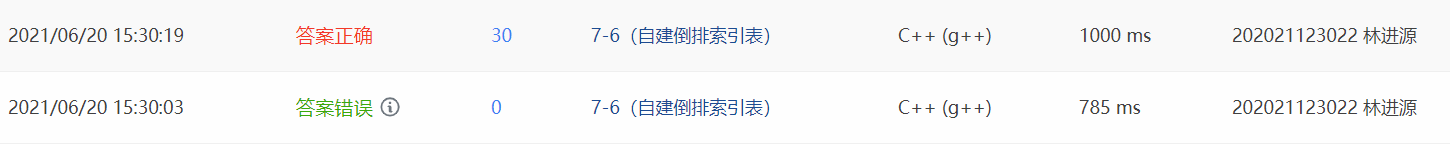### 2.3.3本题知识点

map相关知识和运用、map与矩阵相联立解题

posted @ 2021-06-14 19:27  稶郗  阅读(31)  评论(0编辑  收藏  举报